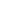Original research

# Detection and comparison of Diabetic Glaucoma using K-means Algorithm and Thresholding Algorithm

## Abstract

Aim: The aim of this research work is for the Innovative Diabetic Glaucoma Detection using modern algorithms and comparing the peak signal to noise ratio (PSNR) between K-means Algorithm and Thresholding Algorithm. Materials and Methods: The sample images were taken from Kaggle’s website. Samples were considered as (N=24) for K-Means Algorithm and (N=24) for Thresholding Algorithm in accordance with total sample size calculated using clinicalc.com by keeping alpha error threshold value 0.05, enrollment rati as 0.1, 95% confidence interval, G power as 80%. The PSNR was calculated by using the MATLAB Programming with standard datasets. Results: Comparison of PSNR is done by independent sample t-test using SPSS software. There is a statistical significant difference between the K-Means Algorithm and Thresholding Algorithm with p= 0.001, p<0.005 (PSNR = 12.6320) showed better results as compared to the K-Means Algorithm (PSNR = 9.8375). Conclusion: Thresholding Algorithm was found to give a higher PSNR than in K-Means Algorithm in the Innovative Diabetic Glaucoma Detection.

## Imprint

Farheen Naz, Jenila Rani D. Detection and comparison of Diabetic Glaucoma using K-means Algorithm and Thresholding Algorithm. Cardiometry; Issue 25; December 2022; p.858-864; DOI: 10.18137/cardiometry.2022.25.858864; Available from: https://www.cardiometry.net/issues/no25-december-2022/diabetic-glaucoma-k-means-algorithm

## Keywords

Innovative Diabetic Glaucoma Detection,  Machine Learning,  K-Means Algorithm,  Thresholding Algorithm,  MATLAB Programming,  Peak Signal to Noise Ratio (PSNR)Current issueCardiometry's libraryFounders of Cardiometry